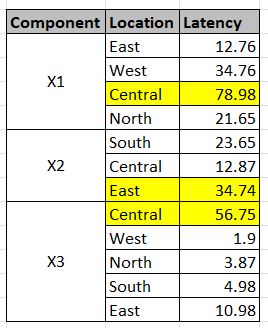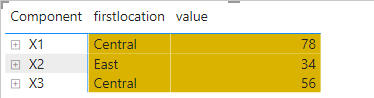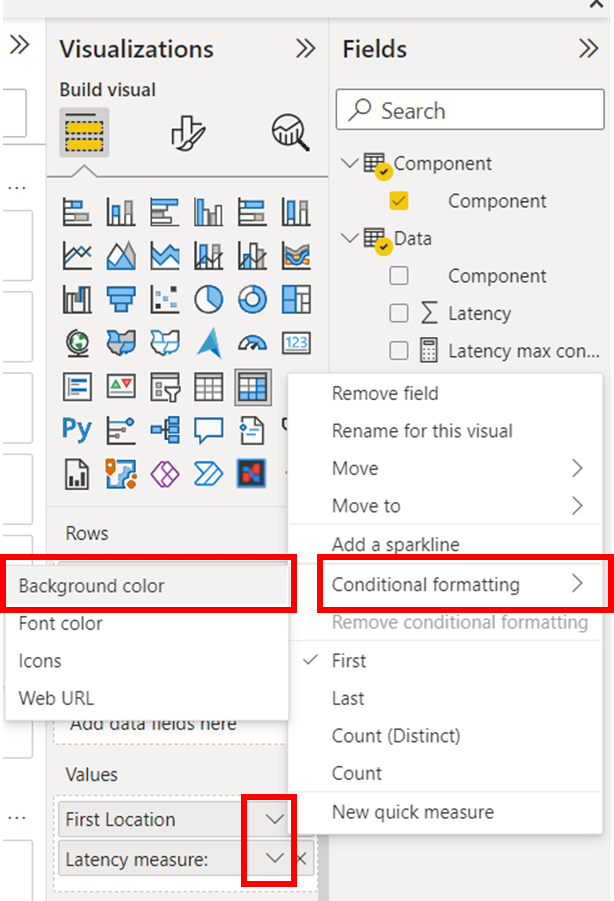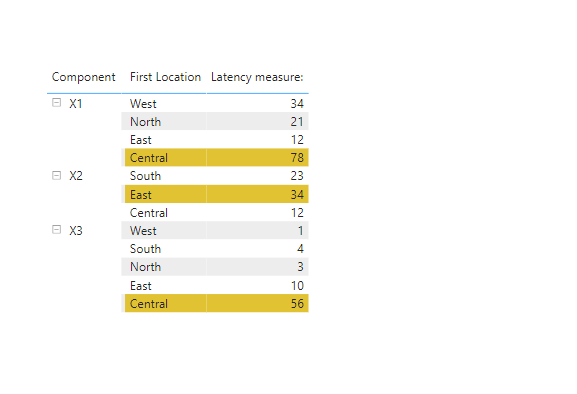cancel
Showing results for
Did you mean:

Fabric is Generally Available. Browse Fabric Presentations. Work towards your Fabric certification with the Cloud Skills Challenge.Microsoft

## Highlight rows in a matrix table with a maximum value

I'm using a dataset as shown below. It contains the latency of different components across the locations.I'm using a matrix table with [Component] and [Location] field in Rows and [Latency] in Values. While the rows are collapsed, I can display the max value from the list of rows under each component. As an example, X1 has [12.76,34.76,78.98,21.65] and maximum value 78.98 is displayed. I'm able to display it using the MAX of latency. PFA snapshot with the stepped layout view.Now I would like to highlight the row with the maximum value for each component. The expected visual while expanded is shown below1 ACCEPTED SOLUTIONCommunity Support

Hi, @subhendude ;

You could create measures as follow:

``````firstlocation =
IF(ISINSCOPE('Location'[Location]),
MAX('Data'[Location]),
CALCULATE(MAX('Data'[Location]),FILTER('Data',
[Latency]=CALCULATE(MAX('Data'[Latency]),ALLEXCEPT('Data','Data'[Component])))))``````
``````value =
IF(ISINSCOPE('Location'[Location]),
MAX('Data'[Latency]),
CALCULATE(MAX('Data'[Latency]),FILTER(ALL('Data'),[Component]=MAX('Component'[Component]))))``````
``````condition =
VAR _max =
CALCULATE ( MAX ( Data[Latency] ), FILTER(ALL('Data'),[Component]=MAX('Component'[Component])))
return IF([value]=_max,1)``````

The final show:Best Regards,
Community Support Team _ Yalan Wu
If this post helps, then please consider Accept it as the solution to help the other members find it more quickly.

4 REPLIES 4Community Support

Hi, @subhendude ;

You could create measures as follow:

``````firstlocation =
IF(ISINSCOPE('Location'[Location]),
MAX('Data'[Location]),
CALCULATE(MAX('Data'[Location]),FILTER('Data',
[Latency]=CALCULATE(MAX('Data'[Latency]),ALLEXCEPT('Data','Data'[Component])))))``````
``````value =
IF(ISINSCOPE('Location'[Location]),
MAX('Data'[Latency]),
CALCULATE(MAX('Data'[Latency]),FILTER(ALL('Data'),[Component]=MAX('Component'[Component]))))``````
``````condition =
VAR _max =
CALCULATE ( MAX ( Data[Latency] ), FILTER(ALL('Data'),[Component]=MAX('Component'[Component])))
return IF([value]=_max,1)``````

The final show:Best Regards,
Community Support Team _ Yalan Wu
If this post helps, then please consider Accept it as the solution to help the other members find it more quickly.Super User

Hi,

Please try writing a measure like below, and put it into the background color conditional formatting.

``````Latency measure: =
SUM( Data[Latency] )``````

``````Latency max condition: =
VAR _max =
CALCULATE ( MAX ( Data[Latency] ), ALL ( Location[Location] ) )
RETURN
IF ( [Latency measure:] = _max, 1 )
``````

Please check the below pictures and the attached pbix file.If this post helps, then please consider accepting it as the solution to help other members find it faster, and give a big thumbs up.Microsoft

Thanks for the solution. It worked except a scenario...

I saw you're using SUM for Latency measure. If I change it to MAX, the rows appeared as yellow highlighted while collapsed.

The behavior I'm looking is to display max of latency while collapsed and highlight the row with the max value while expanded.Super User

@subhendude , You can only highlight the value, not row column, Assume latency  is a meausre

Create a measure

if(calculate(maxx(Summarize(Table,Table[Compnent], Table[location]), Table[latency]), filter(allselected(Table), Table[Compnent] = max(Table[Compnent]) && Table[location] = max(Table[location])  )) = [latency], "Yellow", "white")

use in conditional formatting using field value option

How to do conditional formatting by measure and apply it on pie?Announcements#### Power BI Monthly Update - November 2023

Check out the November 2023 Power BI update to learn about new features.#### The largest Power BI and Fabric virtual conference

130+ sessions, 130+ speakers, Product managers, MVPs, and experts. All about Power BI and Fabric. Attend online or watch the recordings.Top Solution Authors
Top Kudoed Authors
Users online (6,098)